150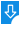10
2585

# Parallel integer multiplier (4x4 bits)  (1.0) Free Download

Parallel integer multiplier (4x4 bits) 1.0 Description:
Parallel integer multiplier (4x4 bits) is an application that simulates the highly regular structure of an integer array multiplier. The multiplier used in the application takes two 4-bit inputs X and Y and generates the 8-bit product value P. Parallel integer multiplier (4x4 bits) is developed using the Java programming language and can be run on Mac OS X, Windows and Linux.Parallel integer multiplier (4x4 bits): Parallel integer multiplier (4x4 bits) for Mac, Simulates the regular structure of an integer
Publisher: University of HamburgDownload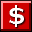Buy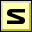Screenshot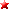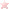Rate it
Category: Math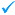Publisher: University of Hamburg    More titles >>
Last Updated: 01/14/2019
Requirements: Mac OS X